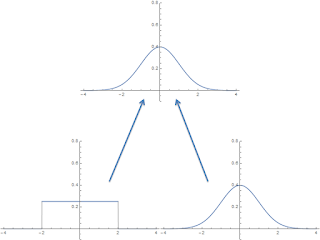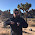Thursday, April 14, 2016

Neo-Fisherism and causality

Stephen Williamson has a new blog post about neo-Fisherism. Let me first say I think I am coming around to the idea that the New Keynesian model really can have a neo-Fisher solution. You guessed correctly there's a "but" coming. However, first let me show why I don't think it's a weird conclusion.

Let's take a model where the output gap is dependent on (a functional of) expected future inflation

$$y_{t} \left[ E_{t} \pi_{t+\Delta t} \right] = H(\pi_{t}, ... , \pi_{t+\Delta t}^{M}, ...)$$

where $M$ represents some model of expectations. Let's re-write this assuming the model of future expectations depends on the actual value of inflation in the future:

\begin{align} y_{t} \left[ E_{t} \pi_{t+\Delta t} \right] &= H(\pi_{t}, ... , \pi_{t+\Delta t}^{M}, ...)\\ &= H(\pi_{t}, ... , \pi_{t+\Delta t}^{M}(\pi_{t+\Delta t}), ...)\\ &= H(\pi_{t+\Delta t}, ...)\\ & \approx h_{0} + h_{1} \pi_{t+\Delta t} \end{align}

where we do a Taylor expansion assuming the deviation from some constant reference inflation value (that gets subsumed into $h_{0}$) is small. This is the functional form that Williamson shows in his blog post (with $h_{0} = 0$ and $h_{1} = 1/b$)

$$y_{\infty} = \frac{1}{b}(R - r) = \frac{1}{b} \pi_{\infty}$$

Basically, if inflation expectations in the future have anything to do with the actual value of inflation in the future, something like the neo-Fisher result can follow.

Now comes that aforementioned "but". But first, as I've looked into before, we need to find out a little bit more about that $E_{t}$ operator. Let me rewrite the operator in terms of a time translation ($\phi$)  and a composition and move $E_{t}$ to the other side:

\begin{align} E_{t} \; f(\pi_{t+\Delta t}) & = f(\pi_{t})\\ E_{t} \; f \circ \phi^{\Delta t}(\pi_{t}) & = f(\pi_{t})\\ f \circ \phi^{\Delta t}(\pi_{t}) & = \left[ E_{t} \right]^{-1} f(\pi_{t})\\ & = U^{\Delta t} f(\pi_{t}) \end{align}

Where we make the identification

$$U^{\Delta t} \equiv \left[ E_{t}\right]^{-1}$$

This means the expectations operator is an inverse Koopman operator, which is to say it propagates functions backwards in time (a normal Koopman operator evolves a dynamical system forward in time). This doesn't mean it propagates the actual future backward in time, but rather represents a consistent way to connect the expected future with the observed present.

This gets at some the causality weirdness of expectations -- where expectations at infinity (critical to the neo-Fisher phenomenon) propagate from the (expected) future into the present (see here or here). It occurs in Woodford's neo-Fisher paper (discussed by Noah Smith and Nick Rowe has a good explanation). In another example of the future propagating into the present, here is Scott Sumner.

Now $E_{t}$ translates unobservable perceived information about the future into present observables. That means it translates some future distribution of possible inflation values into some distribution of present inflation values. Typically, the neo-Fisher argument uses rational expectations and so only cares about the mean, but this misses something critical.

Let's say the distribution of future inflation is a normal distribution with some mean and variance. Because of the central limit theorem, the sum of a large number of random variables drawn from any distribution with the same mean and variance will result in a normal distribution. That is to say there are a lot of different present distributions from which the cumulative inflation time series path (think Brownian motion path) could be drawn that is consistent with a future normal distribution.

Here'a picture where the original distribution is normal and one where it is uniform -- both can lead to a normal distribution (the normal distribution is the defining member of a universality class of distributions with a given mean and variance):The central limit theorem in picture form.

Now what does that backward time translation (i.e. expectations) operator do? Well, it should translate the information in the future normal distribution into the present distribution -- but there are an infinite number of different present distributions consistent with a future normal distribution. How do you choose? You can't. In a sense, the neo-Fisher solution requires you to un-mix the cream and the coffee!Source.

It makes sense if you just look at the mean. Since the future distribution mean depends only on the present distribution mean (the law of large numbers), the present distributions all have the same defining property (i.e. a given mean). However, the real PDFs can be very different -- and you can't see the information loss problem if you ignore them.

Basically, the neo-Fisher result is just a demonstration of the existence of a path that leads to a scenario where interest rates go up and inflation goes up. However, as expectations change in the short run, and the different random walks corresponding to the different present distributions are the most different in the short run, it becomes highly unlikely that the actual path the economy follows will be the neo-Fisher path.

...

Update 15 April 2016

I thought I'd add this picture of the central limit theorem in action from Wikipedia instead of just linking to it:

1.I love that coffee cup entropy picture! I've used that myself on a few occasions. I first saw Sean Carroll present it. I was showing it to people at a St. Patrick's day party when they were pouring black & tan's (kind of ironic for St. Patrick's day... I think the Black & Tan's were the the English backed adversaries of the Irish in 1916)... but anyway, as you can imagine I was the life of the party ... ("Did you hear that colossal bore droning on about entropy?"). =)

1.In looking at it more closely, I think it is actually a Kahlua and cream shot -- ha!

2.That's actually right... Carroll mentioned that it was actually liquor in his talk.

3.Nice post too BTW.

4.... and that guy (w/ the circuit sim) has a bunch more stuff on his website (mostly physics related):
Unfortunately you need to have a "Java enabled browser" to see most of it in action. He gives a link for the plugin, but it's not clear to me which plugin I need (for Firefox)... and Chrome sounds like they dropped support... it's probably opening a whole can of worms... but I would like to see his other stuff!

2.".....assuming the model of future expectations depends on the actual value of inflation in the future...."

How can expectations of future inflation be a function of actual future inflation?

It's illogical.

1.Welcome to the strange world of rational expectations :)

In that model of expectations:

E[πt+1] = πt+1 + εt+1

with ε having zero mean. Therefore

E[πt] = H(πt+1)

I am not here to defend the idea of rational expectations. It's just that the neo-Fisher model assumes rational expectations, and if you follow the rest of the argument I present you can see that creates a contradiction.

My personal opinion is that this shows rational expectations (or any expectations that have future macroeconomic values in them) are wrong. However, as presented, I've stuck to the more limited conclusion that rational expectations at infinity (or simply far in the future) are wrong.

3.Inflation in Brazil is 10% and the rate on 10 year gov bonds is 5%. Why is the neofisherian result a surprise? Why doesn't neo fisherism make sense in such a scenario? Until real rates are positive it seems totally logical to me it's what i'd expect.

1.First, that is just the Fisher equation (i = π + r, which is fine), not the neo-Fisher result Williamson is talking about which is about the output gap and zero (or low) interest rates depressing inflation.

As I said at the top of the post, I do think if you look at the mean values, rational expectations does deliver the neo-Fisher result.

However, the issue is that the neo-Fisher result does not conserve information (you can destroy information, but the neo-Fisher result creates information which is not allowed -- I can't figure out the original distribution from the normal distribution resulting from the central limit theorem).

Check out this picture:

https://en.wikipedia.org/wiki/Central_limit_theorem#/media/File:Central_limit_thm.png